## 题目描述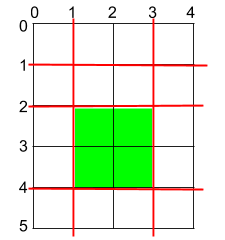输入：h = 5, w = 4, horizontalCuts = [1,2,4], verticalCuts = [1,3]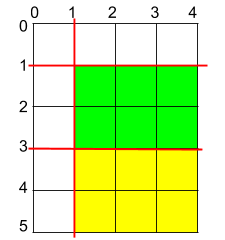输入：h = 5, w = 4, horizontalCuts = [3,1], verticalCuts = 



输入：h = 5, w = 4, horizontalCuts = , verticalCuts = 



2 <= h, w <= 109
1 <= horizontalCuts.length < min(h, 10
5)
1 <= verticalCuts.length < min(w, 10^5)
1 <= horizontalCuts[i] < h
1 <= verticalCuts[i] < w

## 解法

JAVA

class Solution {
public int maxArea(int h, int w, int[] horizontalCuts, int[] verticalCuts) {
Arrays.sort(horizontalCuts);
Arrays.sort(verticalCuts);
int mod = 1000000007;
int hSize = horizontalCuts.length;
int wSize = verticalCuts.length;
long maxM = h - horizontalCuts[hSize - 1];
long maxN = w - verticalCuts[wSize - 1];
maxM = Math.max(maxM,horizontalCuts);
maxN = Math.max(maxN,verticalCuts);
for (int i = 1; i <hSize; i ++) {
maxM = Math.max(maxM,horizontalCuts[i] - horizontalCuts[i-1] );
}
for (int i = 1; i <wSize; i ++) {
maxN = Math.max(maxN,verticalCuts[i] - verticalCuts[i-1] );
}
return  (int)((maxM * maxN ) % mod);
}
}


CPP

class Solution {
public:
static int maxArea(int h, int w, vector<int> &horizontalCuts, vector<int> &verticalCuts) {
//排序
sort(horizontalCuts.begin(), horizontalCuts.end());
sort(verticalCuts.begin(), verticalCuts.end());
int hSize = horizontalCuts.size();
int wSize = verticalCuts.size();
int maxH = max(horizontalCuts, h - horizontalCuts[hSize - 1]);
int maxW = max(verticalCuts, w - verticalCuts[wSize - 1]);
for (int i = 1; i < hSize; i++) {
maxH = max(maxH, horizontalCuts[i] - horizontalCuts[i - 1]);
}
for (int i = 1; i < wSize; i++) {
maxW = max(maxW, verticalCuts[i] - verticalCuts[i - 1]);
}
int model = 1000000007;
return int64_t(maxH) * int64_t(maxW) % model;
}

};


## 解题思路

### 横向观察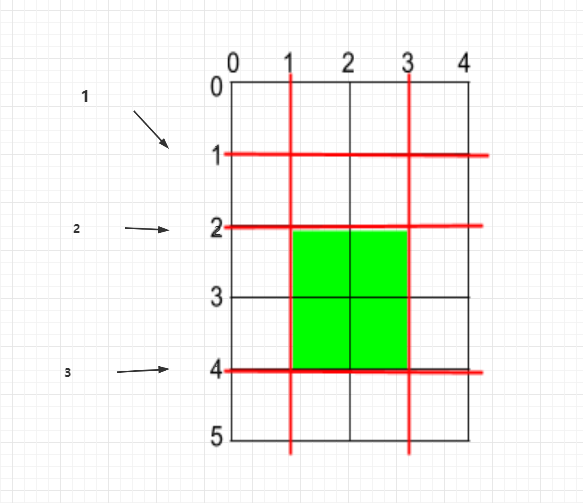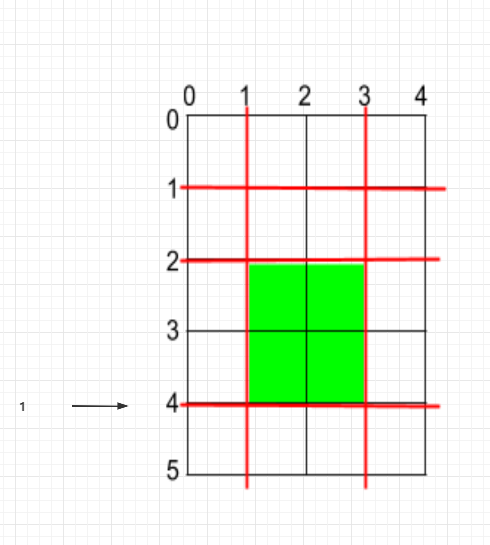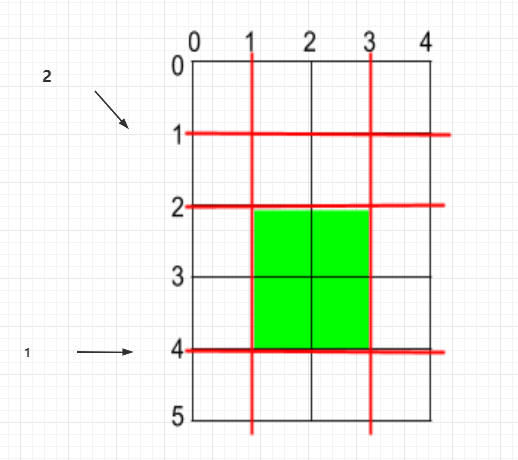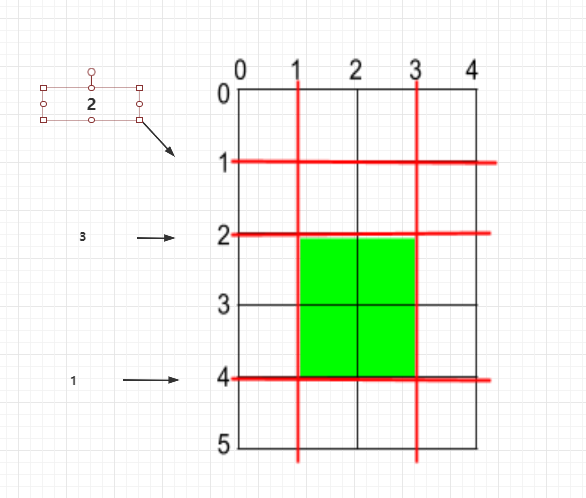int hSize = horizontalCuts.length;
long maxM = h - horizontalCuts[m-1];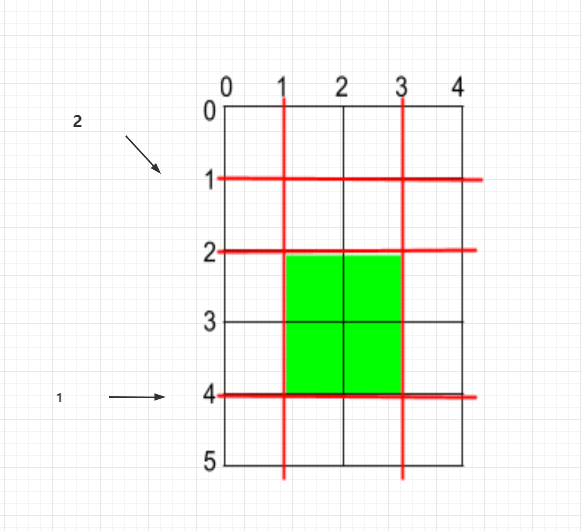maxM = Math.max(maxM,horizontalCuts);


//从1开始遍历
for (int i = 1; i <hSize; i ++) {
maxM = Math.max(maxM,horizontalCuts[i] - horizontalCuts[i-1] );
}


### 纵向

int wSize = verticalCuts.length;
//先切最大的那刀
long maxN = w - verticalCuts[wSize - 1];
maxN = Math.max(maxN,verticalCuts);
for (int i = 1; i <wSize; i ++) {
maxN = Math.max(maxN,verticalCuts[i] - verticalCuts[i-1] );
}